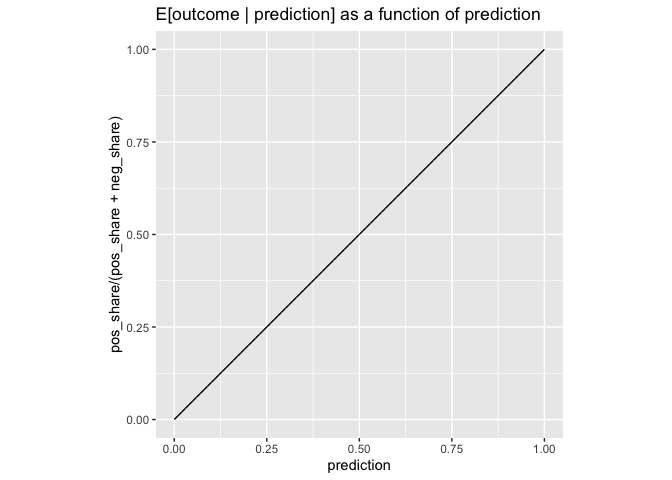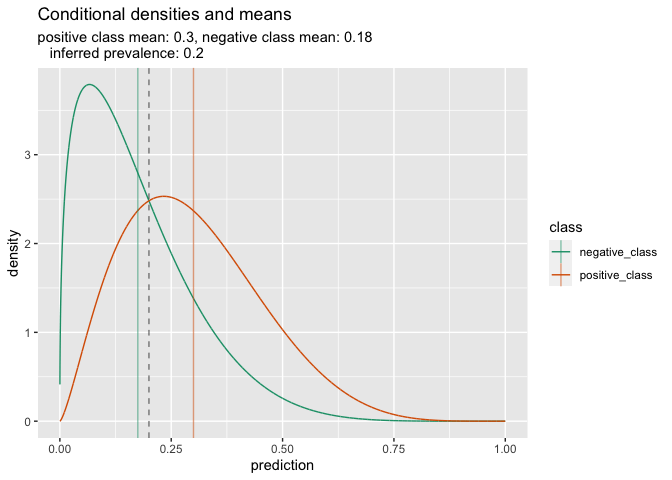# A Single Parameter Family Characterizing Probability Model Performance

## Introduction

We’ve been writing on the distribution density shapes expected for probability models in ROC (receiver operator characteristic) plots, double density plots, and normal/logit-normal densities frameworks. I thought I would re-approach the issue with a specific family of examples.

### Definitions

Let’s define a “probability model” as a model that returns predicted probabilities. Essentially this means the model returns numbers between zero and one, and the model is a good probability model if these predictions obey additional conditions (such as being large on positive examples, small on negative examples, obeying the probability axioms, and even balance conditions).

We will call a probability model fully calibrated if we have `E[outcome | prediction] = prediction` for all observed values of `prediction`. We note, not all calibrated models are fully calibrated.

## Our Example

Any fully calibrated probability model’s performance can be characterized as follows.

We assume an unconditioned distribution of the model predictions: `density(p)`. Then the distribution of predictions on positive truth-value examples must be a shape of the form `c p density(p)` for some constant `c`, and the distributions of the predictions conditioned to come from the negative truth-value examples must be of the form `d (1 - p) density(p)` for some constant `d`. Our point is: being fully calibrated is a very strong condition. In this situation knowing the unconditioned distribution of predictions and the positive outcome prevalence determines the two conditional prediction distributions.

Let’s work a very interesting specific example of the above. Let’s take our unconditional distribution of model predictions to be Beta distributed with shape parameters `a` and `b`. Then some algebra allows us to derive the fully balanced condition implies that the distribution of the predictions on known positive outcomes must be Beta distributed with parameters `(a + 1, b)`, and the distribution of the predictions on the known negative outcomes must be Beta distributed with parameters `(a, b + 1)`.

By the methods of an earlier note we know the the `prevalence` must obey the equation:

```   prevalence E[prediction | positive] +
(1 - prevalence) E[prediction | negative] = prevalence
```

Substituting in the known expected values for the conditional Beta distributions we have:

```   prevalence (a + 1) / (a + b + 1) +
(1 - prevalence) a / (a + b + 1) = prevalence
```

Which implies `prevalence = a / (a + b)`. This prevalence is also the mean of the unconditional distribution and the unique point where the two conditional densities cross (this is confirmed by some algebra on the Beta densities).

### Examining an Instance

Let’s see an instance of this example in `R`.

``````library(ggplot2)
library(WVPlots)``````

We pick an example prevalence.

``````prevalence <- .2
# a / (a + b) = prevalence
# so b = a * (1-prevalence) / prevalence
# and a = b * prevalence / (1 - prevalence)``````

This leaves us one degree of freedom remaining, let’s use this to set `a` to `1.4`.

``````a <- 1.4
b <- a * (1 - prevalence) / prevalence ``````

Confirm `a / (a + b)` matches the specified prevalence.

``a / (a + b)``
``##  0.2``

## Demonstrating the Relations

### Basic Summaries and Densities

Let’s look at all three of the distributions of interest: the unconditional distribution of predictions, the distribution of predictions on known positive examples, and the distribution of examples on known negative examples.

``````step <- 0.0001
prediction <- seq(step, 1 - step, step)

d_mutual <- dbeta(prediction, shape1 = a, shape2 = b)
d_pos <- dbeta(prediction, shape1 = a + 1, shape2 = b)
d_neg <- dbeta(prediction, shape1 = a, shape2 = b + 1)``````

The conditional densities weighted by what fraction of the system they are should add up to the unconditional density. That is easy to confirm quantitatively.

``````d_pos_share <- d_pos * prevalence
d_neg_share <- d_neg * (1 - prevalence)

error <- max(abs((d_pos_share + d_neg_share) - d_mutual))
error``````
``##  2.220446e-15``

With a bit more work we can depict this graphically.

``````pf <- data.frame(
prediction = prediction,
share = d_pos_share,
density = d_pos,
class = 'positive_class',
stringsAsFactors = FALSE)
nf <- data.frame(
prediction = prediction,
share = d_neg_share,
density = d_neg,
class = 'negative_class',
stringsAsFactors = FALSE)
uf <- data.frame(
prediction = prediction,
share = d_mutual,
density = d_mutual,
class = 'unconditioned',
stringsAsFactors = FALSE
)
cf <- data.frame(
prediction = prediction,
share = d_pos_share + d_neg_share,
density = d_pos_share + d_neg_share,
class = 'combined',
stringsAsFactors = FALSE
)

rf <- data.frame(
prediction = prediction,
pos_share = d_pos_share,
pos_density = d_pos,
neg_share = d_neg_share,
neg_density = d_neg,
combined_share = d_pos_share + d_neg_share,
mutual_density = d_mutual,
stringsAsFactors = FALSE)``````
``````lf <- rbind(pf, nf)
plot_lim <- 0.7
lf <- lf[lf\$prediction <= plot_lim, ]
ggplot() +
geom_line(
data = lf,
mapping = aes(x = prediction, y = share, color = class)) +
geom_ribbon(
data = cf[cf\$prediction <= plot_lim, ],
mapping = aes(x = prediction, ymin = 0, ymax = share),
alpha = 0.3
) +
geom_line(
data = uf[uf\$prediction <= plot_lim, ],
mapping = aes(x = prediction, y = share),
linetype = 3
) +
scale_color_brewer(palette = "Dark2") +
ggtitle("Unconditioned distribution decomposed into positive and negative contributions")``````Note the conditioned curves are the densities re-scaled by to integrate to their fraction of contribution, this moves the crossing point away from the prevalence.

### Full Calibration

We can also confirm the the depicted model score is fully calibrated. We are looking for the following line to match the line `y = x`.

``````ggplot(
data = rf,
mapping = aes(x = prediction, y = pos_share/(pos_share + neg_share))) +
geom_line() +
coord_fixed() +
ggtitle("E[outcome | prediction] as a function of prediction")``````### Conditional Summaries

The per-class means and prevalences can be confirmed as follows.

``````means <- data.frame(
mean = c(sum(pf\$density * pf\$prediction) / sum(pf\$density),
sum(nf\$density * nf\$prediction) / sum(nf\$density)),
class_prevalence = c(prevalence, 1 - prevalence),
class = c('positive_class', 'negative_class'),
stringsAsFactors = FALSE)

knitr::kable(means)``````
mean class_prevalence class
0.3000000 0.2 positive_class
0.1750018 0.8 negative_class
``````recovered_prev <- sum(means\$class_prevalence * means\$mean)
recovered_prev``````
``##  0.2000014``

The conditional curves cross where `beta(a, b+1)(x) = beta(a+1, b)(x)`. This is at `x = 1/(1 + beta(a, b+1)/beta(a+1, b))`, which is also `a / (a + b)`.

``````crossing_prediction <- 1/(1 + beta(a, b+1)/beta(a+1, b))

print(crossing_prediction - a / (a + b))``````
``##  2.775558e-17``
``````print(
dbeta(crossing_prediction, a, b + 1) -
dbeta(crossing_prediction, a + 1, b))``````
``##  -5.329071e-15``

### A Graphical Summary

All of the above relations can be summarized in the following annotated graph.

``````ggplot() +
geom_line(
data = rbind(pf, nf),
mapping = aes(x = prediction, y = density, color = class)) +
geom_vline(
data = means,
mapping = aes(xintercept = mean, color = class), alpha = 0.5) +
geom_vline(xintercept = recovered_prev, alpha = 0.5, linetype = 2) +
scale_color_brewer(palette = "Dark2") +
ggtitle("Conditional densities and means",
subtitle = paste0(
"positive class mean: ",
format(means\$mean[means\$class == 'positive_class'], digits = 2),
", negative class mean: ",
format(means\$mean[means\$class == 'negative_class'], digits = 2),
"\n   inferred prevalence: ", format(recovered_prev, digits = 2)
))``````## As an ROC Plot

And we can, of course, represent the model performance as an ROC plot.

``````rf <- data.frame(
prediction = pf\$prediction,
Sensitivity = 1 - cumsum(pf\$density)/sum(pf\$density),
Specificity = cumsum(nf\$density)/sum(nf\$density))

ggplot(
data = rf,
aes(x = 1 - Specificity, y = Sensitivity)) +
geom_line() +
geom_ribbon(aes(ymin = 0, ymax = Sensitivity), alpha = 0.5) +
coord_fixed() +
ggtitle("ROC plot of model score")``````## Some Limiting Cases

For a given prevalence situation the prediction densities of all fully calibrated models that have an unconditional beta distribution are given by shape parameters:

• `(c a, c b)` for the unconditional distribution of predictions.
• `(c a + 1, c b)` for the distribution of the prediction on positive examples.
• `(c a, c b + 1)` for the distribution of the prediction on negative examples.

Where `(a, b)` are positive numbers such that `prevalence = a / (a + b)` and `c` is an arbitrary positive constant.

For this family of model performances the limit as we take `c` to positive infinity is the constant model that always predicts the prevalence. This is an oblivious or uninformative model, but it is fully calibrated on its single limiting prediction.

If we take the limit of `c` to zero from above, then the limiting model is an impulse at zero of perfect negative predictions and an impulse at one of perfect positive predictions.

For intermediate values of `c` we get models that are fully calibrated, match the observed prevalence, and have varying degrees of quality for their predictions.

If `a = b`, then the previously discussed uniform distribution example is part of the model family.

## Conclusion

For a given problem prevalence, the set of fully calibrated model performance densities that have an unconditional Beta distribution form a single parameter family. When model performance is characterized by a single parameter of this nature, then using a single derived parameter such as AUC (area under the curve) becomes a proper model comparison procedure in that dominant model quality is ordered the same way as the score. (Please see ref, though this is not the model characterization family we anticipated there; also a different characterization than normal or logit normal scoring; some notes on the asymmetries in this formulation can be found here.)

Categories: Pragmatic Data Science### jmount

Data Scientist and trainer at Win Vector LLC. One of the authors of Practical Data Science with R.

1.jmount says: